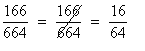# 1603. Sir Bedavere’s Bogus Division Solutions

The wise Sir Bedavere often uses non-standard logic, yet achieves positive results . Well, it seems he has been at it again, this time with division. He has determined that canceling the common digit of a numerator and denominator produces the correct answer. Of course, Sir Bedavere only tried this on a small sample of three digit numbers. An example of what he did is shown in the following division problem (in which he canceled the common 6):Your task is to find all three digit number combinations with the following property:

number combinations where removing the rightmost digit from the top number (numerator) and the identical leftmost digit from the bottom number (denominator) leaves the result of the calculation unchanged.

Omit all of the trivial cases ― xxx/xxx = xx/xx (222/222 = 22/22). The solutions are to be shown in increasing order of the top number (the numerator).

### 输入格式

NONE! There is no input for this problem.

### 输出格式

Show the bogus division problems one to a line in the format shown below (which gives a sample merely to show the format) ― single spaces separate the non-blank characters.

217 / 775 = 21 / 75

249 / 996 = 24 / 96

### 样例

Input
NONE

Output
NONE


61 人解决，71 人已尝试。

75 份提交通过，共有 257 份提交。

3.6 EMB 奖励。